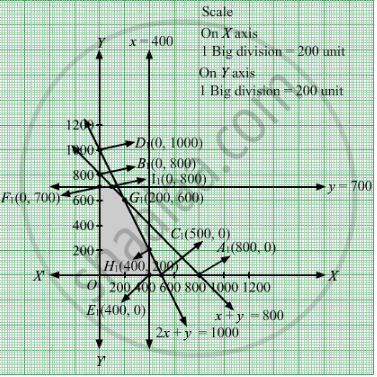Share
Notifications

View all notifications
Books Shortlist
Your shortlist is empty

# A Company Produces Two Types of Leather Belts, Say Type a and B. Belt a is a Superior Quality and Belt B is of a Lower Quality. Profits on Each Type of Belt Are Rs 2 and Rs 1.50 per Belt, Respectively - CBSE (Arts) Class 12 - Mathematics

Login
Create free account

Forgot password?
ConceptGraphical Method of Solving Linear Programming Problems

#### Question

A company produces two types of leather belts, say type A and B. Belt A is a superior quality and belt B is of a lower quality. Profits on each type of belt are Rs 2 and Rs 1.50 per belt, respectively. Each belt of type A requires twice as much time as required by a belt of type B. If all belts were of type B, the company could produce 1000 belts per day. But the supply of leather is sufficient only for 800 belts per day (both A and B combined). Belt A requires a fancy buckle and only 400 fancy buckles are available for this per day. For belt of type B, only 700 buckles are available per day.
How should the company manufacture the two types of belts in order to have a maximum overall profit?

#### Solution

Let the company produces x belts of type A and y belts of type B.
Number of belts cannot be negative.
Therefore,

$x, y \geq 0$ It is given that leather is sufficient only for 800 belts per day (both A and B combined). Therefore,
x + y ≤ 800

It is given that the rate of production of belts of type B is 1000 per day.Hence, the time taken to produce y belts of type B is $\frac{y}{1000}$ And, since each belt of type A requires twice as much time as a belt of type B, the rate of production of belts of type A is 500 per day and therefore, total time taken to produce x belts of type A is $\frac{x}{500}$ Thus, we have $\frac{x}{500} + \frac{y}{1000} \leq 1$
$\Rightarrow 2x + y \leq 1000$
Belt A requires a fancy buckle and only 400 fancy buckles are available for this per day. x ≤ 400
For belt of type B, only 700 buckles are available per day.
≤ 700
Profits on each type of belt are Rs 2 and Rs 1.50 per belt, respectively. Therefore, profit gained on x belts of type A and y belts of type B is Rs 2x and
Rs 1.50y respectively.Hence, the total profit would be Rs (2x + 1.50y).
Let Z denote the total profit.
$\therefore$ z =  $2x + 1 . 5y$
Thus, the mathematical formulat​ion of the given linear programming problem is  Max Z =  $2x + 1 . 5y$ subject to $x + y \leq 800$
$2x + y \leq 1000$
$x \leq 400$
$y \leq 700$
$x, y \geq 0$

First we will convert inequations into equations as follows :
x + y = 800, 2x + y = 1000, x = 400, y = 700, x = 0 and y = 0

Region represented by x + y ≤ 800:
The line x + y = 800 meets the coordinate axes at A1(800, 0) and B1(0, 800) respectively. By joining these points we obtain the line x + y = 800. Clearly (0,0) satisfies the x + y = 800. So, the region which contains the origin represents the solution set of the inequation x + y ≤ 800.

Region represented by 2x + y ≤ 1000:
The line 2x + y = 1000 meets the coordinate axes at C1(500, 0) and D1(0, 1000) respectively. By joining these points we obtain the line 2x + y = 1000. Clearly (0,0) satisfies the inequation 2x + y ≤ 1000. So,the region which contains the origin represents the solution set of the inequation 2x + y ≤ 1000.
Region represented by x ≤ 400:
The line x = 400 will pass through E1(400, 0). The region to the left of the line  x = 400 will satisfy the inequation x ≤ 400.
Region represented by y ≤ 700:
The line y = 700 will pass through F1(0, 700). The region below the line y = 700 will satisfy the inequation y ≤ 700.
Region represented by x ≥ 0 and y ≥ 0:
Since, every point in the first quadrant satisfies these inequations. So, the first quadrant is the region represented by the inequations x ≥ 0, and ≥ 0.
The feasible region determined by the system of constraints x + y ≤ 800, 2x + y ≤ 1000, x≤ 400, y ≤ 700, x ≥ 0, and y ≥ 0 are as follows.
The feasible region determined by the system of constraints isThe corner points are F1(0, 700), G1(200, 600), H1(400, 200) and E1(400, 0).
The values of Z at these corner points are as follows

 Corner point Z= 2x +1.5y F1(0, 700) 1050 G1(200, 600) 1300 H1(400, 200) 1100 E1(400, 0) 800

The maximum value of Z is 1300 which is attained at G1(200, 600).

Thus, the maximum profit is Rs 1300 obtained when 200 belts of type A and 600 belts of type were produced.
Is there an error in this question or solution?

#### Video TutorialsVIEW ALL 

Solution A Company Produces Two Types of Leather Belts, Say Type a and B. Belt a is a Superior Quality and Belt B is of a Lower Quality. Profits on Each Type of Belt Are Rs 2 and Rs 1.50 per Belt, Respectively Concept: Graphical Method of Solving Linear Programming Problems.
S